# Electric Field Physics Gillis Analogy The electric field

• Slides: 15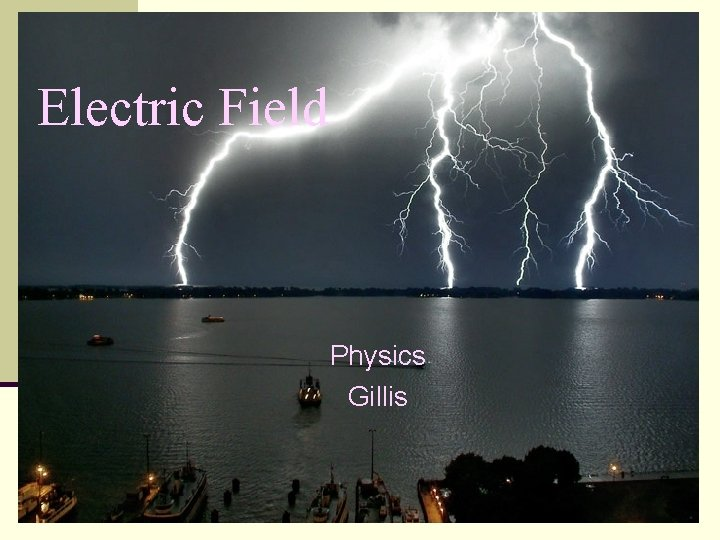Electric Field Physics Gillis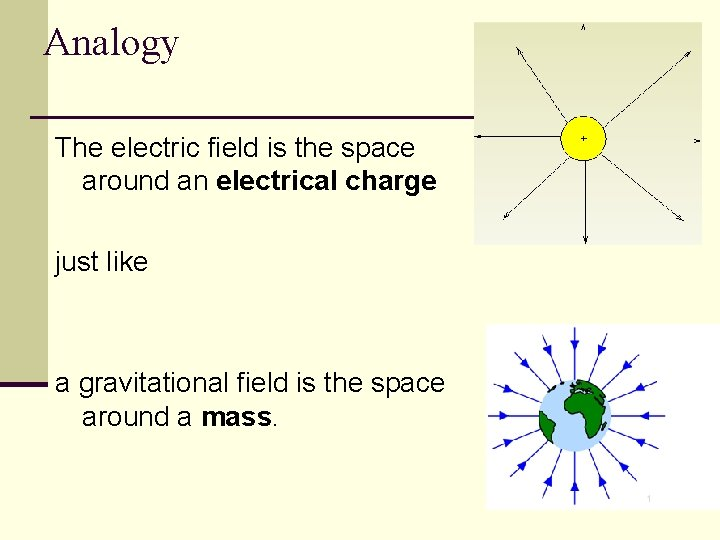Analogy The electric field is the space around an electrical charge just like a gravitational field is the space around a mass.Electric Field n Space around a charge.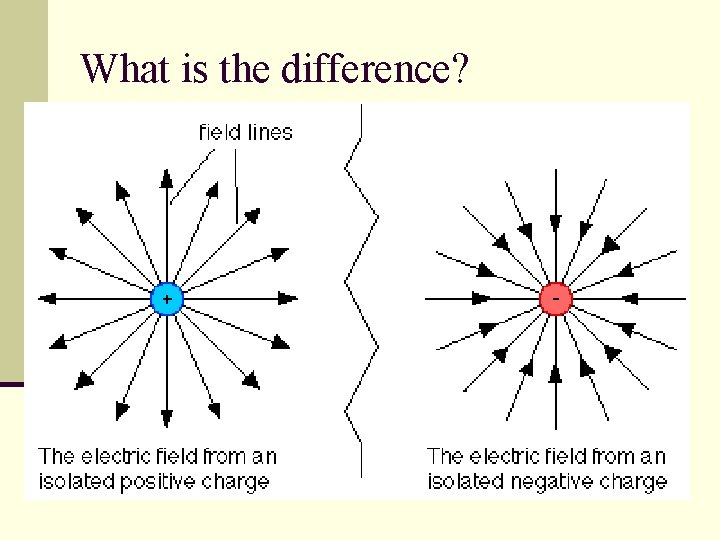What is the difference?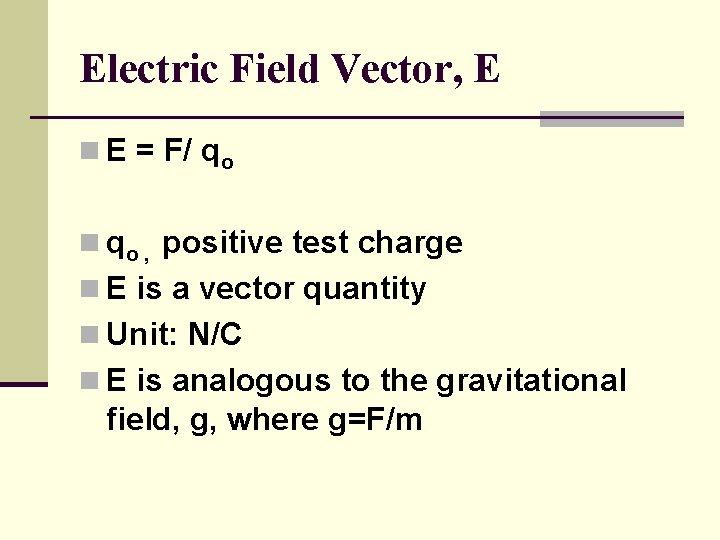Electric Field Vector, E n E = F/ qo n qo , positive test charge n E is a vector quantity n Unit: N/C n E is analogous to the gravitational field, g, where g=F/m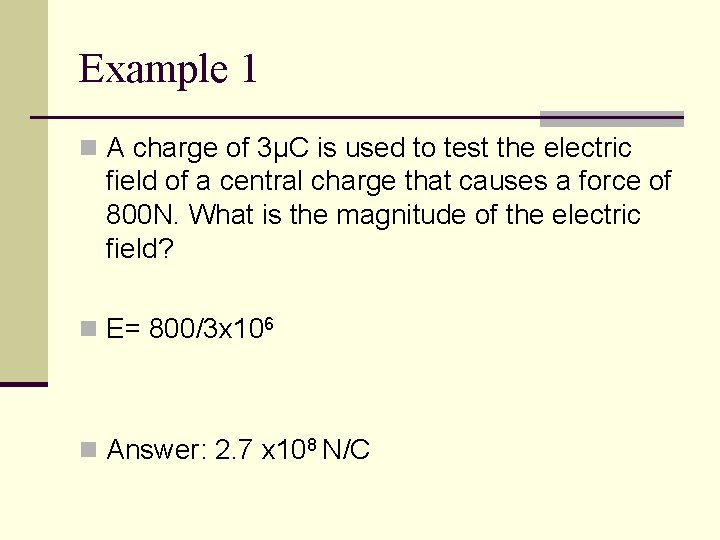Example 1 n A charge of 3µC is used to test the electric field of a central charge that causes a force of 800 N. What is the magnitude of the electric field? n E= 800/3 x 106 n Answer: 2. 7 x 108 N/CElectric Field http: //higheredbcs. wiley. com/legacy/colleg e/halliday/0471320005/simulations 6 e/inde x. htm? newwindow=true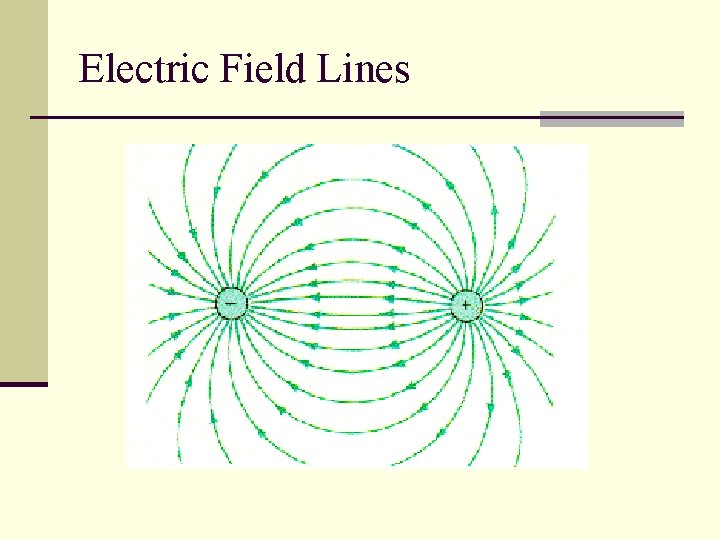Electric Field LinesElectric Field Lines of two Positive Charges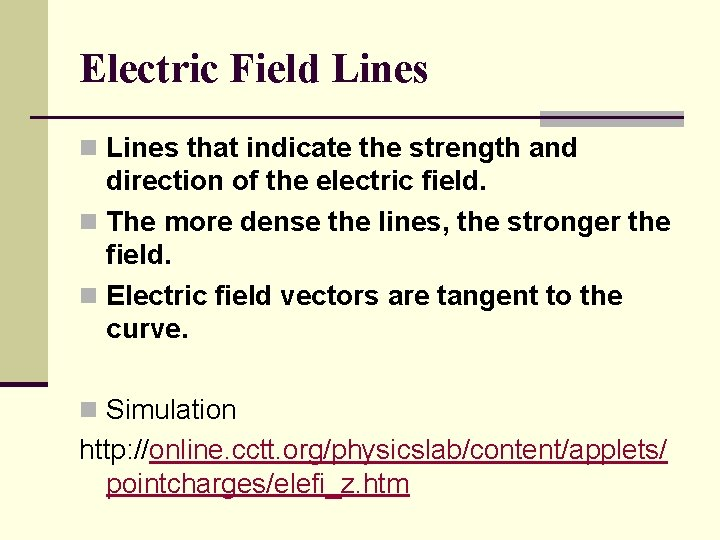Electric Field Lines n Lines that indicate the strength and direction of the electric field. n The more dense the lines, the stronger the field. n Electric field vectors are tangent to the curve. n Simulation http: //online. cctt. org/physicslab/content/applets/ pointcharges/elefi_z. htm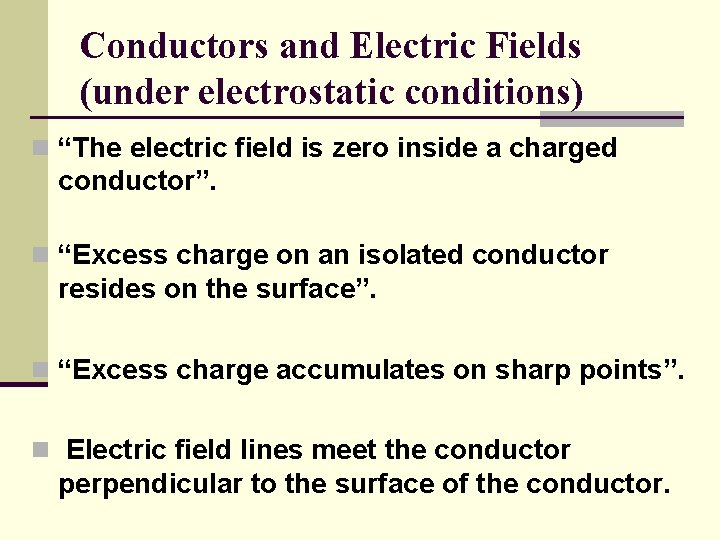Conductors and Electric Fields (under electrostatic conditions) n “The electric field is zero inside a charged conductor”. n “Excess charge on an isolated conductor resides on the surface”. n “Excess charge accumulates on sharp points”. n Electric field lines meet the conductor perpendicular to the surface of the conductor.Shielding n The electric field is zero inside a charged conductor.Which field is stronger? n. A BElectric Field for a Point Charge Using E=F/qo and Coulomb’s Law prove: E=k Q ______ d 2 where Q is the central charge.Example 2 n A test charge of +3µC is located 5 m to the east of a -4µC charge. n A) Find the electric force felt by the test charge. n B) Find the electric field at that location. n Answer: 4. 32 x 10 -3 N, 1. 44 x 103 N/C along the –x axis.